Chapter 11

# Chapter 11

Chapter 11:-

## The Government Functions and Scope.

Plus Two Economics Chapter 11 The Government Functions and Scope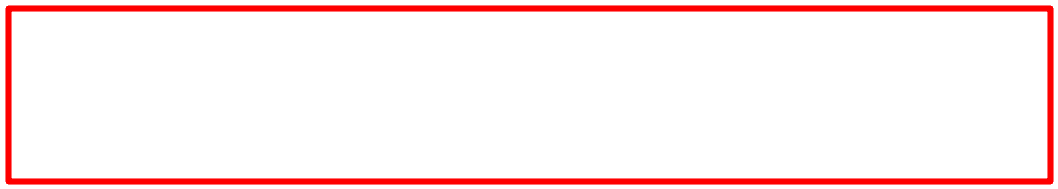## Introduction

In the modern world the government has to perform several responsibilities. Maintenance of law and order, protection of life and property of people, administration of justice, providing basic infrastructure, promotion of public welfare, etc., are the basic functions of the government. In addition to these, the government has to perform three economic functions.

## Functions of Government

The functions of the government are broadly classified into three. They are

#### 3) Stablization function.

They are explained below:

#### 1) Allocation function

Allocation function of the government refers to making available to all, public goods, arranging the resources for the same purpose and distributing them effectively among all the people. It is important to distinguish between public goods and private goods.

Roads, Parks, Street lights, Public administration, Defence, etc., to be used by all are called public goods. No one can be excluded from the consumption of these goods. That is, public goods if used by anyone cannot be denied to another. This implies that consumption of public goods is non-rivalry in character. No price will be demanded for these and so market has no role to play.

Goods like food articles, clothes, consumer durables like television, vehicles, cinema, travel facilities, etc., that we buy from the market for a price are called private goods because they are bought for private use.

So the function of the government to supply public goods to the people is called the Allocation Function.

#### 2) Distribution function

Distribution function refers to the activities of the government to reduce inequality in income and wealth. Market economy is characterized by high inequalities in the distribution of income and wealth. Such social injustice is not good. So, the government intervenes in the redistribution of income. Higher rates of taxes are imposed on the rich. This is called progressive taxation. Thus by imposing higher rates of taxes on the income of the rich, the Government gets revenue which is used to provide food articles at low prices, free education, free health services and social security services to the poor. This function of the government is called Distribution Function.

#### 3) Stablization function.

Stabilization function refers to the measures taken by the government to maintain price stability, economic stability, etc. Economic growth is not linear, it is cyclical. Market economies are prone to periodic fluctuations like booms and recessions. Booms lead to inflation while recessions lead to deflation and unemployment. In such periods of economic expansion and contraction, the government has to intervene to stabilize the economy. The government intervenes in such circumstances using monetary policy and fiscal policy to maintain stability. Deficiency of aggregate demand results in recession. Then the government introduces projects that help to increase public expenditure and increase AD. Conversely, during inflation, the government decreases public expenditure so as to bring down AD thereby controlling price rise. Government intervention of this type is called Stabilization Function.

## Components of Government Budget

Every year the government presents before the Parliament a statement of the estimated receipts and expenditure of the government of India. This is called Budget. It is the annual financial statement of income and expenditure of the government. A budget has two accounts. (I) Revenue account and (II) Capital account.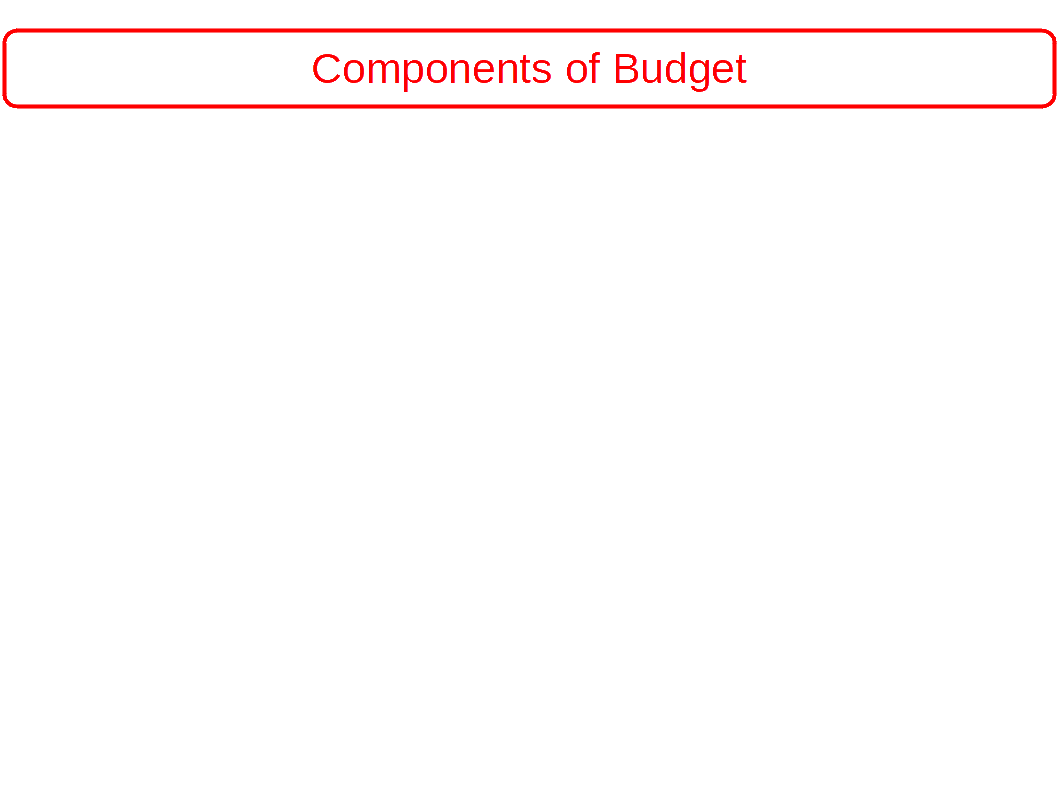## I Revenue Account

Revenue Account or revenue budget consists of revenue receipts and revenue expenditure. The revenue receipts include tax and non-tax receipts which are of recurring nature. For example, revenue receipts like various taxes, fees, fines, etc., are received by the government every year. Similarly, revenue expenditures like interest payments, salaries, subsidies etc., also are of recurring nature.

### 1. Revenue Receipts

The receipts which do not create liabilities or reduce government assets are called revenue receipts. Revenue receipts can be divided into (A) Tax Revenue (B) Non-tax Revenue

#### A. Tax Revenue

Tax is the compulsory payment by the tax payer to the government without any direct benefit or special consideration. Tax is the main source of revenue to the government. Taxes are (a) Direct Taxes (b) Indirect Taxes.

(a) Direct Taxes

Taxes imposed on individuals and institutions are called direct taxes. Income tax, company tax, wealth tax, gift tax, etc., are the important direct taxes.

(b) Indirect Taxes

Taxes imposed on goods and services are called indirect tax, Customs duty, excise duty, service taxes, etc., are the main indirect taxes imposed by the Central government. Sales tax (VAT) is the main indirect tax imposed by the State government.

#### B. Non-tax Revenue

Revenue from sources other than taxes is known as non-tax revenue. The main components of non-tax revenue are explained below:

1. Fees

Fees are compulsory payments made by those who receive direct benefit or service from the government. Examples: Licence fee, court fee, etc.

2. Fines and penalties

Fines and penalties are charges levied for delayed payment or for infringement of some laws. e.g., fine for delayed payment of telephone bills, penalties for violating traffic rules, etc.

3. Special assessments

Special assessments are compulsory contributions made by the people of a particular region in return for certain special benefits derived by them. e.g., Construction of a road in a region increases the market value of land in that area. Special assessments are imposed on the land owners of that region to recover a part of the cost of construction.

4. Income from public property

This is the income earned by the government from public property. e.g., Income from the lease or sale of buildings, quarries, mines, etc.

5. Grants-in-aid

Grants are payments made by one government to another for a particular purpose like a developmental activity. Grants are also given by institutions like the World Bank. In India, the central government gives grants to state governments, who, in turn, give grants to local self governments.

6. Escheats

Property of persons who die with out heirs (successors) goes to the government. This is known as escheats. This is not an important source of revenue to the government.

7. Dividends and Profits

Surpluses (profits) generated by Public sector enterprises are an important source of revenue. e.g., surpluses of departmental undertakings like Railways, P.& T, profits of public sector companies, etc. Dividends on investment made by government are also included.

8. Interest and loans

Interest received on loans granted by the government come under non-tax revenue.

9. Printing of currency

Even though printing of currency cannot be strictly regarded as revenue to the government, it is a fact that it generates income to the government.

### 2. Revenue Expenditure

Expenditure that does not create assets or reduce liabilities is called revenue expenditure. Expenditure like interest payments, salaries and pension, grants-in-aid, subsidies, reliefs, etc., are examples of revenue expenditure.

Revenue expenditure is classified into:

(a) Plan revenue expenditure and

(b) Non-plan revenue expenditure

(a) Plan revenue expenditure

Plan revenue expenditure is the proposed expenditure in the budget for the implementation of Projects and schemes in the plan for the coming year. It relates to

Central plans and

Financial assistance to the Plans of states and union territories.

(b) Non-plan revenue expenditure

Non-plan revenue expenditure is the Proposed expenditure provided in the budget for non-plan activities for the coming year.

This includes,

Interest payments

Defence expenditure

Salaries and pensions

Subsidies

Non-plan grants to states and union territories

Family welfare

Education and health services

Economic services

Natural disaster control and relief

Rural development

## II Capital Account

Capital Account consists of capital receipts and capital expenditure. Capital receipts are those receipts of the government which create liability, or reduce assets of the government. For example, market borrowings create liability while disinvestment reduces financial assets. Capital expenditure is the expenditure of the government which creates physical or financial assets. Buildings, other constructions, plant and machinery, loans to State Governments, etc., are examples for capital expenditure.

### 1. Capital Receipts

Capital receipts are those that create liabilities or reduce the assets of the government. Important sources of capital receipts are the following:

1. Borrowings

2. Recovery of loans and advances

3. Disinvestment

4. Small savings

### 2. Capital Expenditure

Capital expenditure is one which creates assets or reduces liabilities. Expenditure on buildings, plant, machinery, infrastructure, etc., are capital expenditure.

The capital expenditure is classified into:

(a) Plan capital expenditure and

(b) Non-plan capital expenditure

(a) Plan capital expenditure

Capital expenditure is developmental expenditure. It includes:

Capital projects of central plans

Loans to states and union territories for their plans

(b) Non-plan capital expenditure

Non-plan capital expenditure is the proposed expenditure for non-plan activities on a long term basis. It includes

Capital expenditure on defence

Non-defence capital expenditure

Non-plan loans to states and union territories

Other kinds of loans within the country

Loans to foreign governments

### New Initiatives Introduced in 2017-18 Budget

1. The date of budget presentation was advanced to 1st February from the last day of February. This enables early passing of the budget and incurring of the expenditure early in the coming financial year.

2. The Union Budget and the Railway Budget were consolidated into one budget.

3. The distinction between plan expenditure and non-plan expenditure has been abolished.

## Measures of Government Budget Deficit

Budgets are classified into Deficit budget, Balanced budget and Surplus budget.

When the government expenditure is more than revenue, it is called deficit budget. That is, if the anticipated expenditure of the government is more than the anticipated revenue, it is called deficit budget.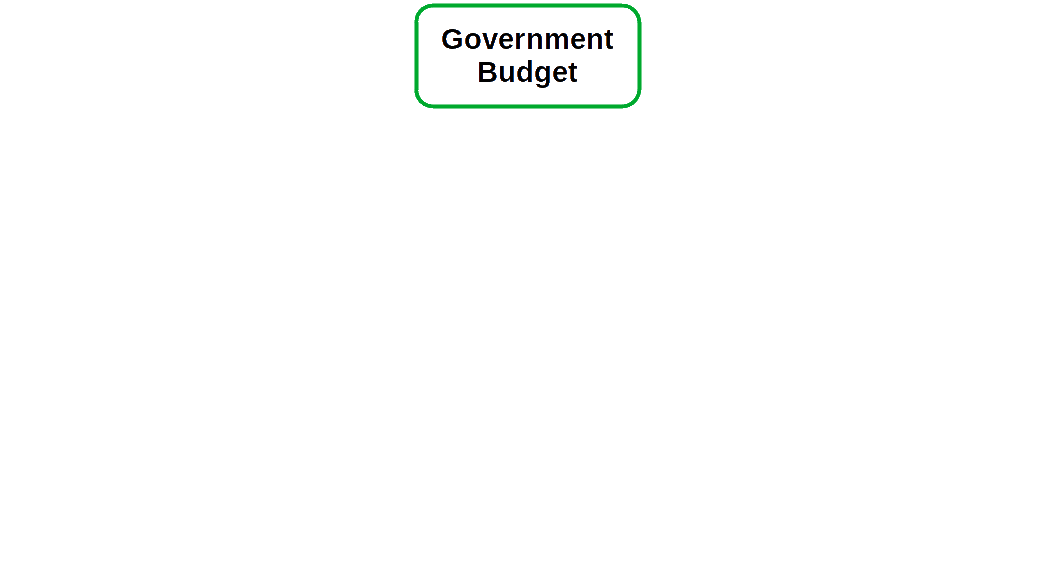###If the anticipated expenditure and anticipated revenue are equal, it is called a balanced budget.

If the anticipated expenditure is less than the anticipated revenue it is called a surplus budget.

Both the deficit budget and the surplus budget are called unbalanced budget.

Different types of budget deficit are,

1. Revenue deficit: If the revenue expenditure is more than revenue receipts of the government it is called revenue deficit. It is the excess of revenue expenditure over revenue receipts.

Revenue deficit = Revenue expenditure – Revenue receipts

2. Fiscal deficit: Fiscal deficit is the excess of total expenditure over total revenue excluding borrowings.

Fiscal deficit = Total expenditure – Total revenue excluding borrowings

Total expenditure includes both revenue expenditure and capital expenditure. But total revenue does not include borrowings.

Gross fiscal deficit = Total expenditure – (revenue receipts + non debt creating – capital receipts)

Fiscal deficit indicates the total requirement of borrowings of the government; So;

Gross fiscal deficit = Net borrowing at home + Borrowing from RBI + Borrowing from abroad.

3. Primary Deficit; Primary deficit is the excess of fiscal deficit over interest payment.

Primary deficit = Fiscal deficit – Interest payment

4. Monetized deficit: Deficit financed by printing currency is called monetized deficit.

• Find 1. Revenue deficit, 2. Fiscal deficit and 3. Primary deficit from the budget details in the table below:

• Table 11.1
Item Rs in crore
Revenue receipts 5773
Capital receipts 4443
Borrowings 4140
Total expenditure 10216
Revenue expenditure 9064
Capital expenditure 1152
Interest payment 2195

1. Revenue deficit = Revenue expenditure – revenue receipt

= 9,064 – 5,773 = ₹ 3,291 crore

2. Fiscal deficit = Total expenditure – (revenue receipts + non-debt creating capital receipts)

Non-debt creating capital receipts = Capital receipts – borrowings

= 4,443 – 4,140 = 303

Fiscal deficit = 10,216 – (5,773 + 303)

= 10,216 – 6,076 = ₹ 4,140 crore

3. Primary deficit = Fiscal deficit – interest payment

= 4,140 – 2,195 = ₹ 1,945 crore

## Fiscal Policy

The policy of the government regarding public expenditure, revenue and borrowing is called fiscal policy. When the economy experiences cyclical fluctuations the government uses fiscal policy to stabilize the economy.

The government can intervene in the economy and achieve stability by using fiscal instruments of taxation and government expenditure. Taxation will reduce the disposable income of the people and thereby reduce their consumption expenditure. If the government does not spend the entire tax revenue this will have the impact of reducing aggregate demand. This policy is implemented during boom and inflation. When the economy is in recession the government takes measures to increase public expenditure. Thus the problems of recession are solved.

## Determination of Equilibrium Income in a Three Sector Economy

Households, firms and the government are the three sectors in the economy. When the government enters the two sector model, it becomes three sector model.

When the government intervenes in the economy, total demand may increase or decrease. It will depend on how much goods and services the government buys, lump-sum tax (T) or proportional tax (tY) and transfer payment (TR). All these variables affect the equilibrium condition of income or output. We shall discuss determination of equilibrium income in a three sector economy through two different circumstances.

(1) Determination of equilibrium income with lump-sum taxes and

(2) Determination of equilibrium income with the imposition of proportional taxes.

#### 1. Determination of Equilibrium Level of Income in case of Lump-sum Taxes

Under three sector economy, AD consists of consumption demand C, investment demand I, and Government demand G. Therefore,

AD in a closed economy with government will be:

$$\mathbf{AD \,=\,C\,+\,I\,+\,G}$$ The different components of Aggregate demand can be briefly described as follows:

#### 1. Consumption demand (C)

Consumption demand depends on the disposable income of the people/households. If we deduct lump-sum tax, (T) from total income and add Transfer payments TR, we get disposable income YD

$$\mathbf{Y^D\,=\,Y\,-\,T\,+\,TR}$$ Whatever may be the income, the fixed amount collected as tax, is known as lump-sum tax. This tax does not depend on income. Lump- sum tax does not affect change in income. Whatever the income, the rate of tax remains the same. Similarly, the Government gives to the households a fixed amount as Transfer Payments. It is supposed that Transfer Payments are fixed (TR = TR). In an economy with the Government, consumption function can be written as

$$\mathbf{C\,=\,f(Y^D)}$$ Consumption demand of the household sector consists of Autonomous Consumption Expenditure and consumption expenditure depending on disposable income. So the consumption equation is

$$\mathbf{C\,=\,\overline{C}\,+\,cY^{D}}$$ Since, YD = Y – T + TR, Consumption demand/expenditure of the economy is C.

$$\mathbf{C\,=\,\overline{C}\,+\,c\,(Y\,-\, T\,+\,\overline{TR})}$$

#### 2. Investment demand (I)

We suppose that investment demand in Three Sector Model is fixed as in Two Sector Model. Therefore,

$$\mathbf{I\,=\,\overline{I}}$$

#### 3. Government demand (G)

In every economy the government buys goods and services. The expenditure on this head is supposed to be fixed. Therefore,

$$\mathbf{G\,=\,\overline{G}}$$ So Aggregate demand is

AD = C + I + G

AD = C + c (Y – T + TR) + I + G

AD = C + cY – cT + cTR + I + G

where,

$$\mathbf{\overline{A}\,=\,\overline{C}\,-\,c\overline{TR}\,+\,\overline{I}\,+\,\overline{G}}$$ In a closed economy the product market reaches equilibrium when the aggregate supply and the aggregate demand of goods and services produced in the economy are equal (AS = Y).

$$\mathbf{Y\,=\,\overline{C}+c(Y-T+\overline{TR})+\overline{I}+\overline{G}}$$ To find equilibrium income, equation should be solved for Y.

Y = C + cY – cT + cTR + I + G

Y – cY = C – cT + cTR + I + G

Y (1 – c) = C – cT + cTR + I + G

If Y* is the equilibrium income,

$$\mathbf{Y^{*}\,=\,\frac{\overline{C}-cT+c\overline{TR}+\overline{I}+\overline{G}}{1-c}}$$ $$or$$ $$\mathbf{\frac{\overline{A}}{1-c}}$$ In the below Diagram the 45° line AS = AD is the equality line. AD = A + cY is the aggregate demand curve. The vertical intercept of AD curve is A and slope is c. At point E, AS is equal to AD. The equilibrium point of income/output is Y*.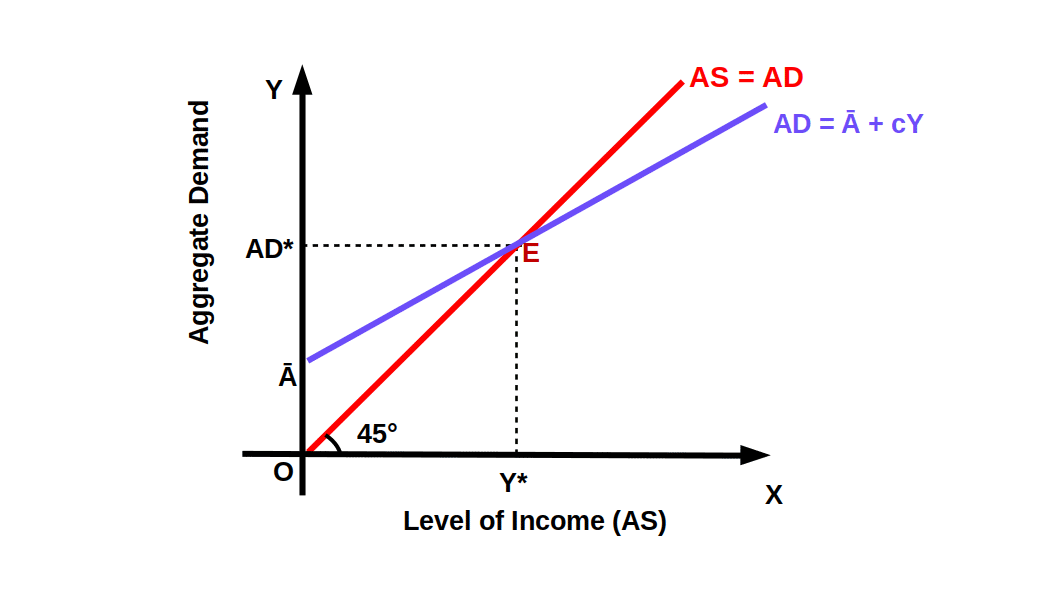Suppose consumption function is C = ₹ 20 + .8YD, I = I ₹ 20, T = T = ₹ 10 TR = TR = ₹ 5, G = G = ₹ 10. Then aggregate demand function

AD = C + I + G

= 20 + .8(Y – 10 + 5) + 20 + 10

= 50 + 8Y – 8 + 4 = 46 + .8Y

Y = 46 + .8Y

Y – .8Y = 46

2Y = 46

Equilibrium income is Y* = $$\frac{46}{.2}$$ = ₹ 230

• In an economy, C is ₹ 100 crore, T is ₹ 10 crore, TR is = ₹ 4 crore, I is ₹ 40 crore, G is ₹ 20 crore, MPC is .75. Find the equilibrium income.

• Equilibrium income is Y*

$${Y^{*}\,=\,\frac{\overline{C}-cT+c\overline{TR}+\overline{I}+\overline{G}}{1-c}}$$

$${=\,\frac{100-.75×10+.75×4+40+20}{1-.75}}$$

$${=\,\frac{160-.75+3}{.25}}$$

$${=\,\frac{155.5}{.25}}$$

= ₹ 622 crore

#### Change in Government Expenditure

Now we shall see how change in government expenditure affects aggregate demand. An increase in government purchases keeping taxes constant, will increase aggregate demand. As a result, line AD will shift upwards from AD1 to AD2. Now, the new equilibrium level shifts from E1 to E2. So, as a result of multiplier effect, equilibrium output increases from Y1* to Y2*, as shown in below given Diagram.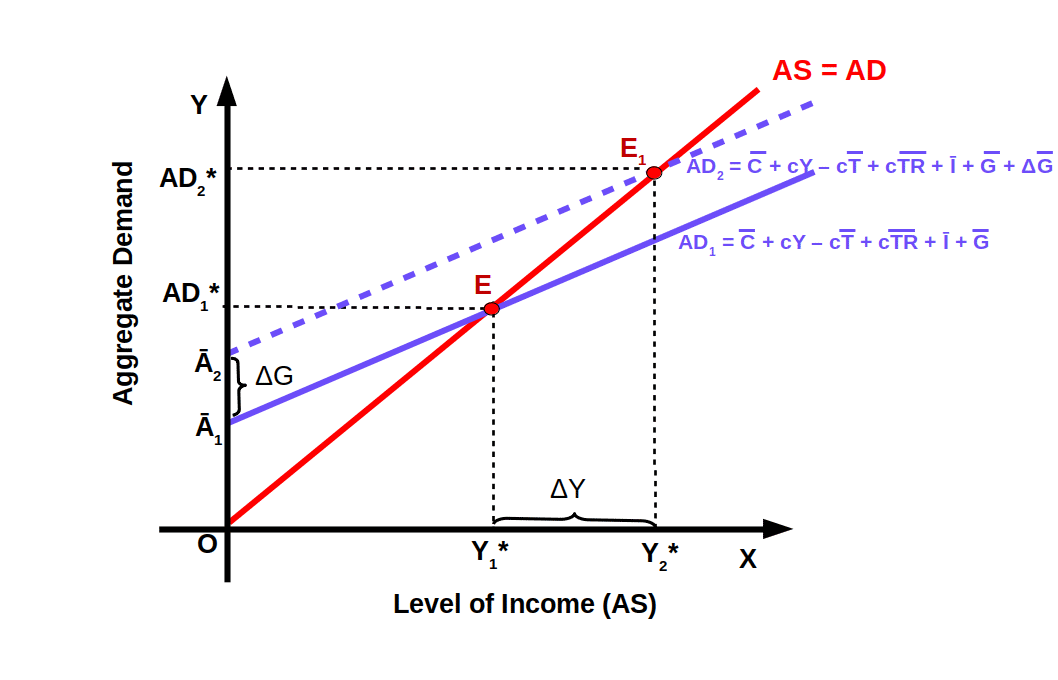#### Government Expenditure Multiplier

Government expenditure multiplier or autonomous government expenditure multiplier is the ratio of change in income (ΔY) to change in government expenditure (ΔG).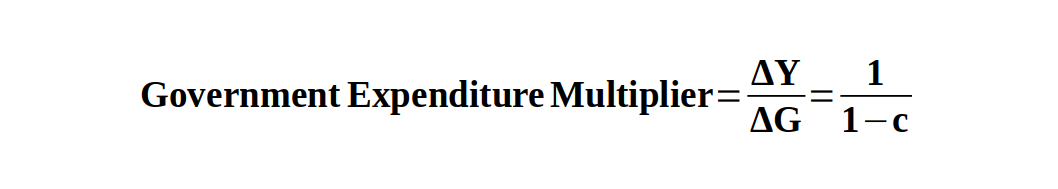• Calculate Government expenditure multiplier if mpc = .8.
• $${\frac{ΔY}{ΔG}}$$ = $${\frac{1}{1-c}}$$

= $${\frac{1}{1-.8}}$$

= $${\frac{1}{.2}}$$

= 5

Government expenditure multiplier is 5; it means that when the government increases its expenditure by one rupee, income increases by ₹ 5.

• When Government expenditure increased from ₹ 100 to ₹ 200, national income increased from ₹ 1,500 to ₹ 1,900. Find the multiplier.
• $${\frac{ΔY}{ΔG}}$$ = $${\frac{400}{100}}$$ = 4

• In an economy with MPC = .75, if government expenditure is increased by ₹ 100, what will be the increase in aggregate income ?
• ΔY = $${\frac{ΔG}{1-MPC}}$$

= $${\frac{100}{1-.75}}$$

= $${\frac{100}{.25}}$$

= 400

#### Changes in Taxes

Changes in taxes affect aggregate demand and equilibrium level of income or output. A cut in taxes increases disposable income at every level. AD curve shifts upwards c times the decrease in taxes. Similarly, when tax is increased AD curve shifts down c times the increase in taxes (cT). This is shown in below Diagram. When tax is reduced by ΔT, income increases by ΔY. At point E1 income is Y1*. As a result of decrease in tax AD curve shifts upwards from AD1 to AD2 Then equilibrium income increases from Y1* to Y2*.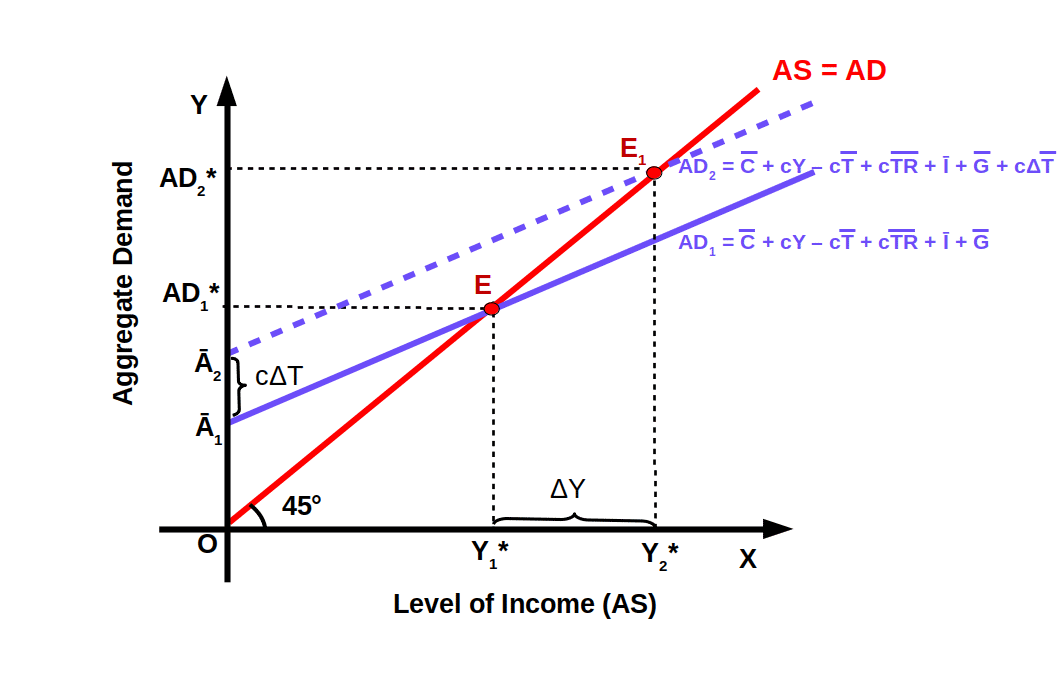#### Tax Multiplier

Tax multiplier is the ratio of change in income (ΔY) to the change in tax (ΔT). Tax multiplier is negative because if we reduce lump-sum-tax, equilibrium income will increase. If lump-sum-tax increases equilibrium income will decrease.

$$\mathbf{Tax\,Multiplier\,=\,\frac{ΔY}{ΔT}\,=\,\frac{-c}{1-c}}$$ Suppose consumption function is C = ₹ 20 + .8YD, I = I ₹ 20, T = T = ₹ 10 TR = TR = ₹ 5, G = G = ₹ 10. Then aggregate demand function

AD = C + I + G

= 20 + .8(Y – 10 + 5) + 20 + 10

= 50 + 8Y – 8 + 4 = 46 + .8Y

Y = 46 + .8Y

Y – .8Y = 46

2Y = 46

Equilibrium income is Y* = $$\frac{46}{.2}$$ = ₹ 230

AD = C + I + G

= 20 + .8(Y – 5 + 5) + 20 + 10

= 50 + 8Y – 4 + 4

= 50 + .8Y

If equilibrium income is Y2*

Y2* = $$\frac{50}{.2}$$ = ₹ 250

In the above mentioned problem equilibrium income was ₹ 230. When Lump-sum-tax was decreased to ₹ 5, equilibrium income increased to ₹ 250. Here increase in income is ₹ 20 (250 – 230 = 20) and decrease in tax is ₹ 5 (10 – 5). Then tax multiplier is 4. That is,

$$Tax\,Multiplier\,=\,\frac{ΔY}{ΔT}$$

= $$\frac{20}{5}$$

= 4

#### Balanced Budget Multiplier

Balanced budget multiplier is the sum of government expenditure multiplier and tax multiplier. That is,

Balanced Budget Multiplier =

$$\mathbf{\frac{ΔY}{ΔG}+\frac{ΔY}{ΔT}}$$ We know that $$\frac{ΔY}{ΔG}$$ = $$\frac{1}{1-c}$$ and

$$\frac{ΔY}{ΔT}$$ = $$\frac{-c}{1-c}$$

So their sum is

$$\frac{ΔY}{ΔG}$$ + $$\frac{ΔY}{ΔT}$$ = $$\frac{1}{1-c}$$ + $$\frac{-c}{1-c}$$

$$\frac{1}{1-c}$$ – $$\frac{c}{1-c}$$ = $$\frac{1-c}{1-c}$$ = 1

The balanced budget multiplier is one which implies, an increase in government expenditure is matched exactly by equal increase in taxes, (G = T). Income will increase by the same amount of the increase in government expenditure.

Suppose that MPC = -8, the government spends all the amount it collects by tax of ₹ 10 (ΔG = ΔT = ₹ 10). Then increase in the income of the people due to government expenditure is ΔY.

ΔY = $$\frac{ΔG}{1-c}$$

= $$\frac{10}{1-,8}$$

= $$\frac{10}{.2}$$

= ₹ 50

When tax of ₹ 10 was imposed, decrease in the people’s income is ΔY.

ΔY = $$\frac{-cΔT}{1-c}$$

= $$\frac{-.8 × 10}{1-.8}$$

= $$\frac{-8}{.2}$$

= ₹ -40

Therefore, net increase in income (50-40) is ₹ 10. This is exactly equal to government expenditure and tax.

#### Transfer Payment Multiplier

Transfer Payment Multiplier is the, ratio of change in income (ΔY), to the change in transfer payment (ΔTR). i.e.,

Transfer Payment Multiplier =

$$\mathbf{\frac{ΔY}{ΔTR}\,=\,\frac{c}{1-c}}$$ Transfer payment multiplier is less than autonomous expenditure multiplier, because a part of any increase in transfer payments becomes saving.

Suppose when TR increases from ₹ 10 to ₹ 15, equilibrium income increases from ₹ 200 to ₹ 230. Then, Transfer Payment Multiplier,$${\frac{ΔY}{ΔTR}\,=\,\frac{30}{5}}$$ = 6. MPC of an economy is .8. Then Transfer Payment Multiplier is $${\frac{ΔY}{ΔTR}\,=\,\frac{c}{1-c}}$$ = $${\frac{.8}{1-.8}\,=\,\frac{.8}{.2}}$$ = 4.

If the MPS of an economy is .25 what is the Transfer Payment Multiplier ?

Since 1- c = MPS,

Transfer payment multiplier is

$${\frac{c}{MPS}\,=\,\frac{.75}{.25}}$$ = 3

#### Proportional Tax and Consumption Function

Proportional tax is the tax that changes with income. The government imposes a fixed percentage of the income as tax. This tax will be varied with the level of income. So in the case of proportional taxes, T becomes tY (T = tY) where t is the rate of tax. So consumption function is

$$C\,=\,C + c(Y – tY + \overline{TR})$$ $$=\,C+cY-ctY+c\overline{TR}$$ $$\mathbf{C\,=\,C+c\overline{TR}+c (1-t)Y}$$ Now we can formulate the AD equation when proportional taxes are imposed. We know that

$$AD\,=\,C+I+G$$ $$AD\,=\,C+c\overline{TR}+c(1-t)Y+\overline{I}+\overline{G}$$ $$AD\,=\,C+c\overline{TR}+\overline{I}+\overline{G}+c(1-t)Y$$ $$\mathbf{AD\,=\,\overline{A}+c(1-t)Y}$$ Here A = C + cTR + i + G, is the sum of all autonomous components. When compared to lump-sum-tax AD, this AD schedule will have a high vertical intercept and smaller slope. In the below given Diagram AD1 is the AD curve, when lump-sum-tax is imposed and AD2 is the AD curve when proportional tax is imposed.

The vertical intercept of AD1 is A1 and c is the slope. The vertical intercept of AD2 is A2 and slope is c (1 – t). It is clear that compared to AD1 , AD2 has a higher intercept and a smaller slope.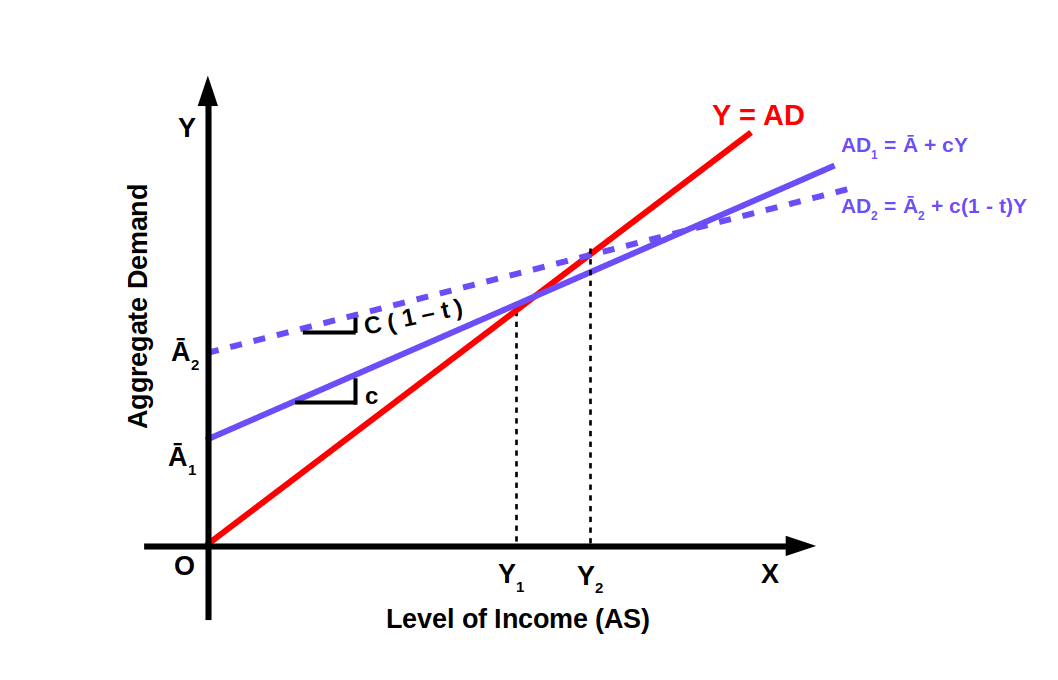#### 2. Determination of Equilibrium Level of Income in case of Proportional Tax

Now we can formulate the equilibrium income equation when proportional taxes are imposed.

AD = C + T + G

= C + c(Y – tY + TR) + I + G

At equilibrium point,

Y = C + c(Y – tY + TR) + I + G

Finally, if equilibrium income is y*

$$\mathbf{Y^{*}\,=\,\frac{\overline{C}+c\overline{TR}+\overline{I}+\overline{G}}{1-c(1-t)}}$$ $$\mathbf{=\,\frac{\overline{A}}{1-c(1-t)}}$$ Since A = C + cTR + i + G in below given diagram. AS = C + I + G is equilibrium line.

AD = A + c(1 -t)Y is the AD curve in case of proportional taxes. At point E, AD and AS are equal. Equilibrium income is Y* and Aggregate demand is AD*.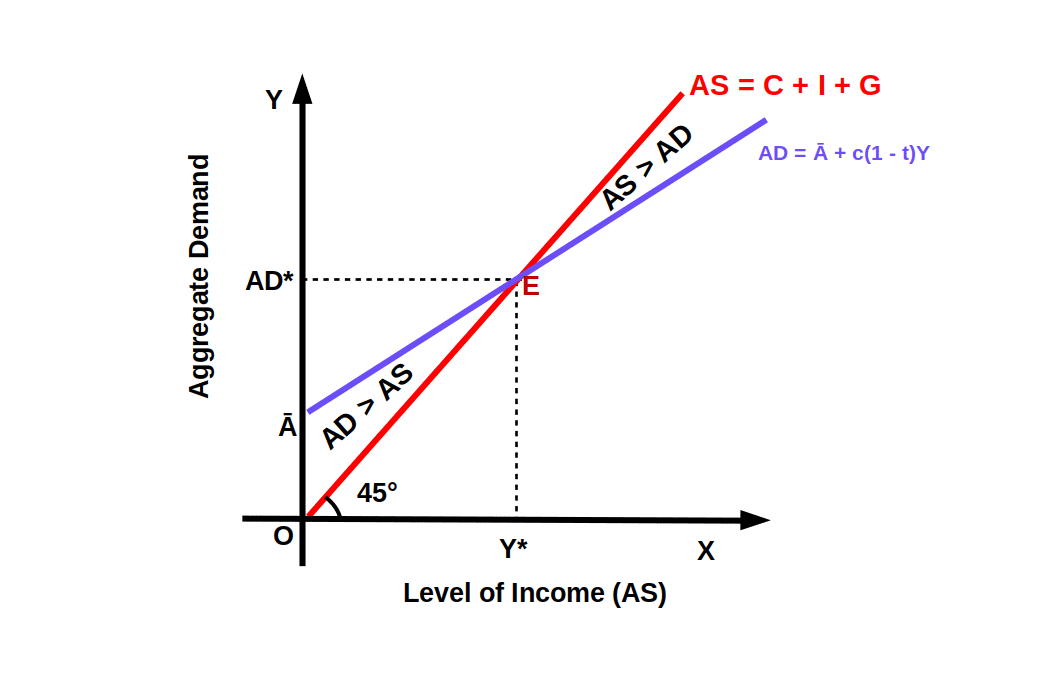Suppose C 20, MPC = .8, t = .1, TR = TR = 5, I = I = 20, G = G = 10. Then equilibrium is

$$Y\,=\,\frac{\overline{A}}{1-c(1-t)}$$ , A = C + cTR + I + G

= $$\frac{20+.8×5+20+10}{1-.8(1-.1) }$$

= $$\frac{50+4}{1-.8×.9) }$$

= $$\frac{54}{1-.72) }$$

= $$\frac{54}{.28) }$$

= 192.86

In equilibrium income the sum of taxes that the Government gets is

T = tY* = 0.1 × 192.86 = 19.29

#### Autonomous Expenditure Multiplier in the Case of Proportional Taxes

When proportional tax is imposed, autonomous expenditure multiplier is

$$\mathbf{\frac{ΔY}{Δ\overline{A}}\,=\,\frac{1}{1-c(1-t)}}$$ In case of proportional tax, autonomous expenditure multiplier is the ratio of change in income (ΔY) to the change in autonomous expenditure (ΔᾹ ) This multiplier depends on MPC and the rate of tax.

If MPC = .8 and t = .1 in the case of proportional tax, autonomous expenditure multiplier is

$$\frac{ΔY}{ΔᾹ }$$

= $$\frac{1}{1-c(1-t) }$$

= $$\frac{1}{1-.8(1-.1) }$$

= $$\frac{1}{1-.8×.9 }$$

= $$\frac{1}{1-.72}$$

= $$\frac{1}{1-.28}$$

= 3.57

A decrease in proportional taxes results in increase in MPC. When proportional taxes decrease AD curve will swing upwards. Similarly, when proportional taxes go up, AD curve swings down. In the below Diagram AD1 is the aggregate demand curve when pro-portional tax is t1. If proportional tax is reduced from t1 to t2 aggregate demand curve will swing upwards from AD to AD2 (t2 < t1). Equilibrium point will move from E1 to E2 and equilibrium income will increase from Y1* to Y2*.The proportional tax was 10% (t =.1) Suppose we decrease it to 8% (t =.08). Then the new equilibrium income Y2* is

Y2* $$\frac{Ᾱ}{1-c(1-t) }$$

= $$\frac{54}{1-.8(1-.08) }$$

= $$\frac{54}{1-.8×.92 }$$

= $$\frac{54}{1-.736}$$

= $$\frac{54}{1-.264}$$

= 204.55

When tax is decreased from t =.1 to t = .08, equilibrium income increases from 192.9 to 204.55.

### Automatic Stabilizer

Policies that function automatically without any conscious action in the economy are called automatic stabilizers. Proportional tax functions as an automatic stabilizer. In the case of proportional tax, income will increase or decrease according to the fluctuations in GDP. Transfer payments given by the government like unemployment pension work as automatic stabilizers. Such automatic stabilizers are called non-discretionary fiscal policy.

### Discretionary Fiscal Policy

The deliberate action in fiscal Policy taken by the government to Stabilise the economy is called discretionary fiscal policy. When the government with the sole objective of achieving economic stability engages in activities like investment and public expenditure, they are called discretionary fiscal policies. Imposing new taxes and increasing public debts are examples.

### Debt

Borrowing of the government to finance the deficit in budget leads to public debt. The government finances deficit through (1) taxation (2) borrowing and (3) printing currency notes. Deficit and debts are closely related. So deficit can be considered a flow to the present accumulated debt. In fact, borrowing leads to accumulation of debt. When the government goes on borrowing, debts accumulate, and increase the interest burden. It imposes burdens on future generations.

### Debt: Different Perspectives

There are two prominent views on government debt. First, whether government debt is a burden. Second, the issue of financing deficit.

When the government goes on borrowing continually it will increase interest payments and impose a burden on the future generations. Today’s borrowings are to be repaid by the future generations. In future the government will have to impose more tax on the income of the people. This will reduce their disposable income. Hence it will reduce future consumption and national income. Consequently the growth of the economy will be impacted. Hence debt becomes a burden on the future generation.

Moreover, in addition to fresh taxes, the government will have to borrow more from the people. Through this, the private sector will have less money for investment. This phenomenon is called crowding out. That is, when the government borrows huge amounts from the financial system, the private sector will experience shortage of funds for investments. So they will get crowded out of the financial markets. As growth and capital investment come down, public debt becomes burden on the future generation.

### Ricardian Equivalence

Another view on debt was put forward by the famous classical economist David Ricardo. According to Ricardo, taxation and borrowing are equal as far as financing the deficit is concerned. Hence the name Ricardian Equivalence. Ricardo believed that when government runs deficit people will save more so that the future generation can repay the debt. The government dissavings (deficit) will be matched by increased savings of the public. Therefore, national savings will remain unaltered. Increased borrowing prepares people for additional taxation in future. Therefore, Ricardo believed that it does not matter whether deficits are financed through borrowing or by raising taxes.

#### Other Perspectives on Deficits and Debt

Budget deficit leads to inflation is is a very important criticism on budget deficit. When the government creases its expenditure, or decreases taxes, aggregate demand will increase. If production units are unable to increase production to meet the increased demand prices rise. But if there are unused resources, they could be used to produce more output, and then increase in AD may not cause inflation.

Budget deficit and debt become unavoidable in times of recession. Even then, the government must be extra vigilant in keeping the deficit under control. If public expenditure is undertaken to provide infrastructure, that is welcome, because it accelerates economic growth. It will lead to high economic growth, higher taxes and income. Through these, repayments of debts become feasible. In this perspective, budget deficit and debt can be justified. At the same time, unlimited debts and uncontrolled deficits are never signs of a healthy economy. Fiscal discipline is a must for economic growth.

### Deficit Reduction

The government can reduce budget deficit by cutting down public expenditure and by imposing fresh taxes. In India, the government tries to reduce the deficit through the following ways:

(1) Making tax collection more effective.

(2) Cutting down wasteful government expenditure.

(3) Selling shares of publi¢ sector undertakings.